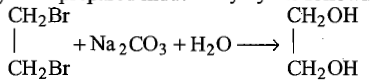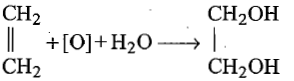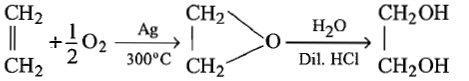The general molecular formula, which represents the homologous series of alkanols is

(a) CnH2nO2        (b) CnH2nO

(c) CnH2n+1O       (d) CnH2n+2O

Concept Questions :-

Alcohols: Preparation, Physical and Chemical Properties
High Yielding Test Series + Question Bank - NEET 2020

Difficulty Level:

$\mathrm{R}-\mathrm{COOH}\to {\mathrm{RCH}}_{2}\mathrm{OH}$. This reduction cannot be done  by:

(a) ${\mathrm{NaBH}}_{4}$                               (b) Na + alcohol

(c) ${\mathrm{LiAlH}}_{4}$                                (d) all of these

Concept Questions :-

Alcohols: Preparation, Physical and Chemical Properties
High Yielding Test Series + Question Bank - NEET 2020

Difficulty Level:

What mass of isobutylene is obtained from 37 g of tertiary butyl alcohol by heating with 20% H2SO4 at 363 K, if the yield is 65%?

1. 16 g

2. 18.2 g

3. 20 g

4. 22 g

Concept Questions :-

Alcohols: Preparation, Physical and Chemical Properties
High Yielding Test Series + Question Bank - NEET 2020

Difficulty Level:

Glycol is prepared industrially by the following reactions:

(a)(b)(c)(d) none of the above

Concept Questions :-

Alcohols: Preparation, Physical and Chemical Properties
High Yielding Test Series + Question Bank - NEET 2020

Difficulty Level:

How many isomers of C5H11OH will be primary alcohols?

1. 5

2. 4

3. 2

4. 3

Concept Questions :-

Alcohols: Preparation, Physical and Chemical Properties
High Yielding Test Series + Question Bank - NEET 2020

Difficulty Level:

Phenol is less soluble in water. It is due to:

1. non-polar nature of phenol

2. acidic nature of -OH group

3. non-polar hydrocarbon part in it

4. none of the above

Concept Questions :-

Phenols: Preparation, Properties, Acidic Nature of Phenol
High Yielding Test Series + Question Bank - NEET 2020

Difficulty Level:

The decreasing order of boiling points of $1°$,$2°$,$3°$ alcohol is:

1. $1°$>$2°$>$3°$

2. $3°$>$2°$>$1°$

3. $2°$>$1°$>$3°$

4. none of these

Concept Questions :-

Alcohols: Preparation, Physical and Chemical Properties
High Yielding Test Series + Question Bank - NEET 2020

Difficulty Level:

The boiling points of thio-ethers are .... than those of ether.

(a) lesser

(b) equal

(c) higher

(d) none of these

Concept Questions :-

Ethers: Preparation, Physical & Chemical Properties, Uses
High Yielding Test Series + Question Bank - NEET 2020

Difficulty Level:

Which reagent is more effective to convert but-2-enal to but-2-enol?

(a) KMnO4                              (b) NaBH4

(c) H2/Pt                                (d) K2Cr2O7/H2SO4

Concept Questions :-

Alcohols: Preparation, Physical and Chemical Properties
High Yielding Test Series + Question Bank - NEET 2020

Difficulty Level:

23g of sodium react with CH3OH to give:

1. 1 mole of O2                           2. 1/2 mole of H2

3. 1 mole of H2                           4. none of these

Concept Questions :-

Alcohols: Preparation, Physical and Chemical Properties